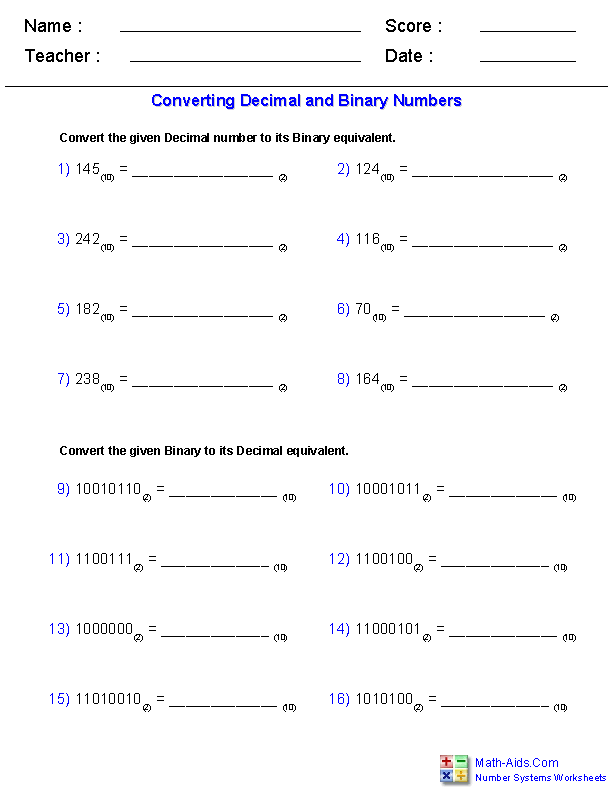# Decimal To Binary Free Test Online### Positive Numbers

#### Decimal to binary conversion of positive numbers

Let's convert the decimal number 99 to binary:
9910 = 1*64 + 1*32 + 0*16 + 0*8 + 0*4 + 1*2 + 1*1 = 1*26 + 1*25 + 0*24 + 0*23 + 0*22 + 1*21 + 1*20.
or 9910 = 11000112
Decimal to binary conversion of positive numbers practice

#### Binary to decimal conversion of positive numbers

Let's convert the binary unsigned number 11001102 from binary to decimal.
Any unsigned binary number bnbn-1...b1b0 can be converted to decimal with the following formula:

bnbn-1...b1b0 = bn×2n + bn-1×2n-1 + ...

+ b0×20,

11001102 = 1×26 + 1×25 + 0×24 + 0*23 + 1*22 + 1×21 + 0*20 = 64 + 32 + 4 + 2 = 102.
Binary to decimal conversion of positive numbers practice

#### Hexadecimal to binary conversion of positive numbers

Convert an integer number from hexadecimal to binary by simply translating each hexadecimal digit into its 4-bit binary equivalent.Hexadecimal numbers have either an 0x prefix, a 16 subscript or an h suffix.
For example, the hexadecimal number 0x9E3 translates into 1001 1110 0011, as the binary values of 9, A and 3 are 1001, 1110 and 0011.
Hexadecimal to binary conversion of positive numbers

#### Binary to hexadecimal conversion of positive numbers

To convert a value from binary to hexadecimal, you first add a number of 0's to the left of the most significant bit of the binary number, so the number of bits of the new binary number is a multiple of 4.

Then, you simply translate each 4-bit binary nibble to its hexadecimal digit equivalent.
Binary to hexadecimal conversion of positive numbers test

#### Hexadecimal to decimal conversion of positive numbers

A hexadecimal number can be converted to decimal by following the method:
0xhnhn-1...h1h0 where hi is any of 0,1,2,3,4,5,6,7,8,9,A,B,C,D,E,F , can be converted to the decimal number:
hn×16n + hn-1×16n-1 + ...+ h1×16 + h0.
Hexadecimal to decimal conversion of positive numbers

#### Decimal to hexadecimal conversion of positive numbers

The simplest way to convert unsigned numbers from decimal to hexadecimal is to transform the number first in binary, as the binary to hex conversion is trivial.
For instance:
9910 = 1*64 + 1*32 + 0*16 + 0*8 + 0*4 + 1*2 + 1*1 = 1*26 + 1*25 + 0*24 + 0*23 + 0*22 + 1*21 + 1*20.

9910 = 11000112 = 0110 00112 = 0x63.
Decimal to hexadecimal conversion of positive numbers

### Signed Integers

#### Decimal to binary conversion of signed numbers

Let's convert to signed binary the following integer: -200
-200 = -256 + 32 +16 + 8 = = -1×28 + 0×27 + 0×26 + 1×25 + 1×24 + 1×23 + 0×22 + 0×21 + 0×20 = 1001110002.
Decimal to binary conversion of signed numbers practice

#### Binary to decimal conversion of signed numbers

Let's convert to decimal the following signed binary number: 10110010
10110010 = -1×27 + 0×26 + 1×25 + 1×24 + 0×23 + 0×22 + 1×21 + 0×20 = -128 + 32 + 16 + 2 = -78.
Binary to decimal conversion of signed numbers

### Positive Fractions

#### Decimal to binary conversion of positive binary fractions

Let's convert to unsigned binary the following positive fraction : 0.6875.
.6875 = 1×0.5 + 1×0.125 + 1×0.0625 = 1×2-1 + 0×2-2 + 1×2-3 + 1×2-4 = 0.1011
Decimal to binary conversion of positive binary fractions

#### Binary to decimal conversion of positive binary fractions

Let's convert to decimal the following unsigned binary fraction: 0.1011
0.1011 = 1×2-1 + 0×2-2 + 1×2-3 + 1×2-4 = .5 + .125 + .0625 = .6875.
Binary to decimal conversion of positive binary fractions

### Signed Binary Fractions

#### Decimal to binary conversion of signed binary fractions

Let's convert to binary the following negative fraction: -0.4375.
-0.4375 = -1 + .5625 = -1 + .5 + .0625 = -1 + 1×2-1 + 0×2-2 + 0×2-3 + 1×2-4 = 1.1001
Decimal to binary conversion of signed binary fractions

#### Binary to decimal conversion of signed binary fractions

Let's convert to decimal the following signed binary fraction: 1.01101.
1.01101 = -1 + 0×2-1 + 1×2-2 + 1×2-3 + 0×2-4 + 1×2-5 = -0.59375.
Binary to decimal conversion of signed binary fractions

### Operations with Binary Numbers

Binary addition is done like adding decimal numbers, except that you have only two digits.

2020 tsx ipo list

You have to remember only that:
0+0 = 0, with no carry,
1+0 = 1, with no carry,
0+1 = 1, with no carry,
1+1 = 0, and you carry a 1.

Let's add the following unsigned binary numbers: 11111 and 10101.Signed numbers in two's complement notation may be added by following these rules:
1.

If the numbers have different signs, they are added as unsigned numbers, and no overflow will occur.
2.

## How To Convert Decimal to Binary

If the numbers have the same sign, they are added and the sign of the resulting number is the same with the sign of both numbers, no overflow occurred.
3. If the numbers have the same sign, they are added and the sign of the resulting number is different than the sign of both numbers, overflow occurred.

#### Unsigned Numbers Multiplication

Multiplication can be done exactly as with decimal numbers, except that you have only two digits.

The only facts to remember are that 0×1=0, and 1×1=1.
Let's multiply the following unsigned binary numbers: 101 and 10.Unsigned Numbers Multiplication